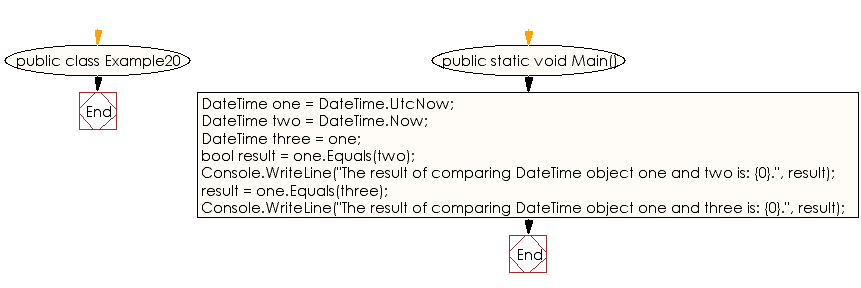﻿ C# - Compare DateTime objects - w3resource# C# Sharp Exercises: Compare DateTime objects

## C# Sharp DateTime: Exercise-20 with Solution

Write a C# Sharp program to compare DateTime objects.

Sample Solution:-

C# Sharp Code:

``````using System;
public class Example20
{
public static void Main()
{
// Create some DateTime objects.
DateTime one = DateTime.UtcNow;

DateTime two = DateTime.Now;

DateTime three = one;

// Compare the DateTime objects and display the results.
bool result = one.Equals(two);

Console.WriteLine("The result of comparing DateTime object one and two is: {0}.", result);

result = one.Equals(three);

Console.WriteLine("The result of comparing DateTime object one and three is: {0}.", result);
}
}
```
```

Sample Output:

```The result of comparing DateTime object one and two is: False.
The result of comparing DateTime object one and three is: True.
```

Flowchart :C# Sharp Code Editor:

Improve this sample solution and post your code through Disqus

What is the difficulty level of this exercise?

Test your Programming skills with w3resource's quiz.

﻿